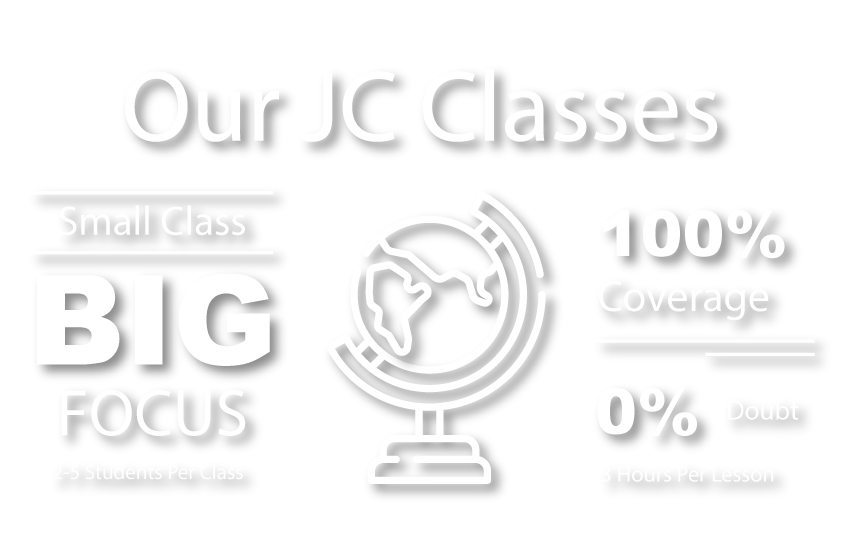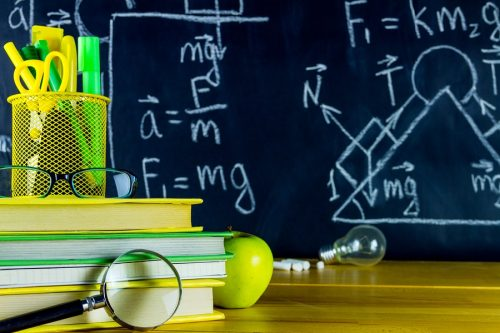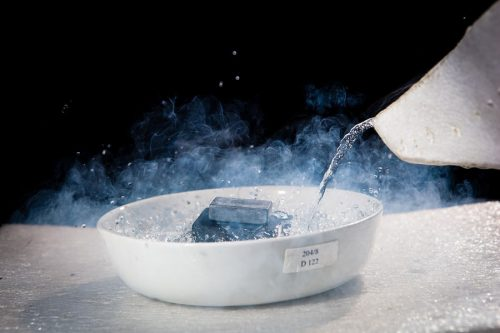# JC H2 Physics Tuition

## JC1 TO JC2 A-LEVEL H2 PHYSICS

At Einstein Education Hub, our other specialization is H2 Physics. Our H2 physics tuition classes in Singapore are specially crafted to keep up to date to the changing JC H2 physics syllabus and examinations. If you need an experienced H2 physics tutor to guide your child through A levels, we are here to help. Get a free H2 physics tuition trial in Singapore with us today.## Our Achievements# 14

YEARS OF EXPERTISE
IN EDUCATION# 100%

OF OUR JC STUDENTS FIND OUR LESSONS SUPER CLEAR AND COMPREHENSIVE# 5

STUDENTS MAXIMUM IN A CLASS. FULL ATTENTION AND GUIDANCE##### Measurement
1. Recall base quantities and their SI units and prefixes
2. Add and subtract coplanar vectors and represent a vector as two perpendicular components
3. Show an understanding of the distinction between systematic errors (including zero error) and random errors, and precision and accuracy
##### Kinematics
1. Use graphical methods to represent distance, displacement, speed, velocity and acceleration
2. Solve problems using equations which represent uniformly accelerated motion in a straight line

##### Dynamics
1. Apply each of Newton’s laws of motion
2. Solve problems using the relationship F = ma
3. Apply the principle of conservation of momentum to solve simple problems

##### Forces
1. Apply Hooke’s law
2. Describe the forces on a mass, charge and current-carrying conductor in gravitational, electric and magnetic fields
3. Qualitative understanding of normal contact forces, frictional forces and viscous forces including air resistance
4. Apply the moment of a force and the torque of a couple
5. Apply the principle of moments to solve related problems
6. Use a vector triangle to represent forces in equilibrium
7. Solve problems using the equation of pressure
8. Calculate the upthrust in terms of the weight of the displaced fluid

##### Work, Energy and Power
1. Calculate the work done in a number of situations including the work done by a gas
2. Derive and use the equation for kinetic energy, gravitational potential energy and elastic potential energy

##### Motion in a Circle
1. Use the concept of angular velocity to solve problems
2. Describe qualitatively motion in a curved path
3. Use centripetal acceleration and centripetal force formulas to solve problems

##### Gravitational Field
1. Recall and use Newton’s law of gravitation
2. Derive and use gravitational field strength of a point mass
3. Derive and use gravitational potential in the field of a point mass
4. Understand geostationary orbits and their applications

##### Temperature and Ideal Gases
1. Explain how empirical evidence leads to the gas laws and to the idea of an absolute scale of temperature
2. Use the equation of state for an ideal gas
3. State the basic assumptions of the kinetic theory of gases
4. Explain how molecular movement causes the pressure exerted by a gas
5. Apply the relationship that the mean kinetic energy of a molecule of an ideal gas is proportional to the thermodynamic temperature##### First Law of Thermodynamics
1. Use the concepts of specific heat capacity and specific latent heat
2. Use the first law of thermodynamics

##### Oscillations
1. Investigate the motion of an oscillator using experimental and graphical methods
2. Define and use equations of simple harmonic motion
3. Describe the interchange between kinetic and potential energy during simple harmonic motion
4. Describe practical examples of damped oscillations, forced oscillations and resonance

##### Superposition
1. Explain and use the principle of superposition
2. Explain the formation of a stationary wave
3. Explain the meaning of the term diffraction
4. Show an understanding of experiments which demonstrate two-source interference
5. Recall and use the Rayleigh criterion

##### Electric Fields
1. Represent an electric field by means of field lines
2. Recall and use Coulomb’s law
3. Describe the effect of a uniform electric field on the motion of charged particles

##### D.C. Circuits
1. Solve problems using the formula for the combined resistance of two or more resistors in series and parallel
2. Solve problems involving series and parallel circuits for one source of e.m.f.

##### Electromagnetism
1. Sketch flux patterns due to currents in a long straight wire, a flat circular coil and a long solenoid
2. Solve problems using the equation for magnetic force
3. Predict the direction of the force on a charge moving in a magnetic field

##### Electromagnetic Induction
1. Calculate magnetic flux and magnetic flux linkage
2. Recall and solve problems using Faraday’s law of electromagnetic induction and Lenz’s law

##### Quantum Physics
1. Show an understanding of the photoelectric effect
2. Explain photoelectric phenomena in terms of photon energy and work function energy
3. Describe and interpret qualitatively the evidence provided by electron diffraction for the wave nature of particles
4. Show an understanding of the existence of discrete electronic energy levels in isolated atoms
5. Explain the origins of the features of a typical X-ray spectrum
6. Show an understanding of and apply Heisenberg position-momentum uncertainty principle